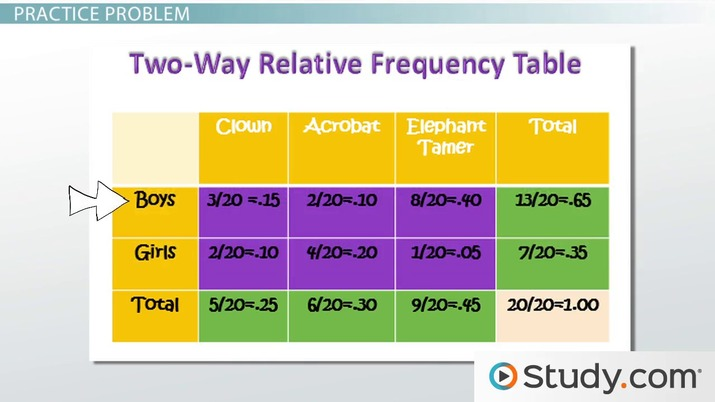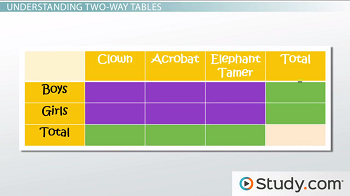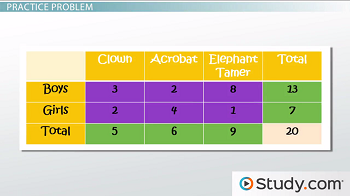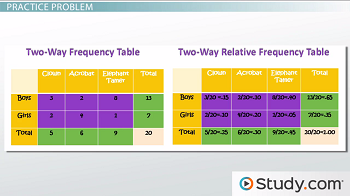# Joint, Marginal & Conditional Frequencies: Definitions, Differences & Examples

Lesson Transcript
Instructor: Cathryn Jackson

Cat has taught a variety of subjects, including communications, mathematics, and technology. Cat has a master's degree in education and is currently working on her Ph.D.

Joint, marginal, and conditional frequencies are all part of analyzing categorical data and two-way tables. This lesson will help you learn the definitions and differences between each concept.

## Joint, Marginal and Conditional Frequencies

Travis is considering running away and joining the circus. He surveys 20 of his friends to determine the most popular career options. He asks his friends, 13 boys and 7 girls, which circus career they would prefer: clown, acrobat, or elephant tamer? Travis uses a two-way table to analyze his data. To do this, he needs to understand joint, marginal, and conditional frequencies.An error occurred trying to load this video.

Try refreshing the page, or contact customer support.

Coming up next: Mathematical Sets: Elements, Intersections & Unions

### You're on a roll. Keep up the good work!

Replay
Your next lesson will play in 10 seconds
• 0:02 Joint, Marginal &…
• 0:29 Understanding Two-Way Tables
• 2:39 Practice Problem
• 8:07 Lesson Summary
Save Save

Want to watch this again later?

Timeline
Autoplay
Autoplay
Speed Speed

## Understanding Two-Way Tables

This is a two-way table - it is a table listing two categorical variables whose values have been paired. Each set of numbers in a two-way table has a specific name.The middle cells are the joint frequency numbers. When analyzing data in a two-way frequency table, you will be looking for joint relative frequency, which is the ratio of the frequency in a particular category and the total number of data values. The purple cells on this table are all joint frequency numbers.

This is called joint frequency because you are joining one variable from the row and one variable from the column. In this example, the row variables are the careers and the column variables are the genders. Therefore, the number of girls that prefer a clown career would be considered a joint frequency.

The numbers in the column on the very right and on the row on the very bottom are the marginal frequency numbers. When analyzing data in a two-way frequency table, you will be looking for marginal relative frequency, which is the ratio of the sum of the joint relative frequency in a row or column and the total number of data values. When you think of marginal frequency, think of the margins on an English paper. The margins are the areas on the edges of the paper, the marginal frequency numbers are the numbers on the edges of a table. On this table, the marginal frequency numbers are in the green cells.

Conditional relative frequency numbers are the ratio of a joint relative frequency and related marginal relative frequency. For example, let's say you wanted to find the percentage of people that selected clown as a career, given those people are girls. You would then find the number of girls that selected clown and divide by the total number of girls in the survey. This is a similar set up to conditional probability, where the limitation, or condition, is preceded by the word given. Let's look at some examples to help you find the different relative frequencies in a two-way table.

## Practice Problem

To analyze data using a two-way table, follow these steps:

1. Create a two-way frequency table
2. Convert your numbers into relative frequency numbers
3. Identify joint and marginal frequencies
4. Calculate conditional relative frequencies

This is a two-way table created using Travis' survey data.The horizontal categorical variable shows the careers and the vertical categorical variable shows the genders. The center numbers, in the purple cells, break down the answers by both categories. The column totals, or the horizontal green cells, show us the total number of responses for each career in the survey.

For example, you can see that out of all of the people surveyed, 9 chose elephant tamer as a career. The row totals, or the vertical green cells, show us the total number of each gender surveyed; we can see that 7 girls and 13 boys answered this survey. The light yellow cell in the bottom right corner is our grand total cell. If you add the green column cells together, you get 20. Likewise, if you add all of the green row cells together, you get 20. That is because there were 20 people surveyed and no one could pick more than one career.

This is a two-way frequency table next to a two-way relative frequency table.In a two-way relative frequency table, each number in the cells is divided by the grand total. That is because we are looking for a percentage that shows us how the data compares to the grand total. For example, the relative frequency of boys that chose clown as a career is .15 or 15%, meaning that 15% of all of the people surveyed were boys and chose clown as a career. Relative frequency shows the relationship between a piece of data and the grand total.

Now that we have converted our numbers into relative frequency numbers, you can identify the joint and marginal relative frequency numbers. Travis wants to know the joint relative frequency of the boys that selected elephant tamer. Can you identify the joint relative frequency number?

Correct! The joint relative frequency is .40 or 40%. Now, can you identify the marginal relative frequency of the people that selected acrobat as a career? Right! The marginal relative frequency is .30 or 30%. Remember, the joint relative frequency numbers are in the purple cells, and the marginal relative frequency numbers are in the green cells.

To unlock this lesson you must be a Study.com Member.

### Register to view this lesson

Are you a student or a teacher?